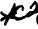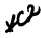S e c t i o n I V . T O R Q U E C O N V E R T E R R E B U I LD cont.
Custom Search*C2 *C2 P A R   8 3 - 8 6 T O R Q U E     C O N V E R T E R     R E B U I LD C H A P    5,   SEC  I V ( 2 )    R e m o v e    1 2    s t a t o r    r o l l e r    b e a r i n gs and   springs   from   the   stator   (fig.   147). ( 3 )    R e m o v e    t h e    r o l l e r    t h r u s t    b e a r i ng a s s e m b l y    f r o m    t h e    f r e e w h e e l    r a c e .    R e m o ve roller   thrust   washer   from   the   stator   (fig.   147). If   either   bearing   or   thrust   washer   is   damaged, replace   both   parts. (4)   Remove   the   front   and   rear   retaining rings,   two   large   thrust   washers   and   two   small t h r u s t    w a s h e r s    f r o m    t h e    s t a t o r    a s s e m b l y . Mark   the   stator   cam   and   stator   assembly   for reference   in   reassembly.   Press   out   the   cam. 8 4 .    C L E A N I NG Refer   to   par.   71   for   cleaning   recommen- d a t i o n s . 8 5 .    I N S P E C T I O N    A N D    R E P A IR R e f e r    t o    p a r .    7 2    f o r    g e n e r a l    i n s p e c t i on and    repair    recommendations.    Repair    and    re- b u i l d    p o i n t s    o f    m e a s u r e m e n t    f o r    f i t s ,    c l e a r- ances   and   wear   limits   are   indicated   by   small, lower   case   letters   in   fig.   373,   fold-out   2.   Re- fer   to   par.   236   for   wear   limits   information. 8 6 .     A S S E M B LY .   T o r q u e    C o n v e r t e r    C o v e r (fig.   373,   fold-out   2) (1)   Install   double-row   ball   bearing   143, retaining   ring   groove   outward,   on   the   hub   of torque   converter   cover   69.   Press   the   bearing until   it   is   firmly   seated   on   the   hub. (2)   Using   spanner   wrench   8351495   (fig. 36),   install   converter   lock   nut   144   (fig.   373, fold-out   2).   Torque   the   nut   to   75   pound-feet. Deform   the   lip   of   the   nut   into   the   two   grooves in   the   hub   of   converter   cover   69. (3)   Install   bearing   support   sleeve   104. Install   the   retaining   ring   on   the   bearing. (4)   Install   the   input   drive   transfer   gear and,   using   a   5/8-inch   wrench,   secure   it   with twenty-four   7/16-20   x   1   self-locking   bolts   (fig. 1 4 2 ) .    T i g h t e n    t h e    b o l t s    t o    6 4 - 7 7    p o u n d - f e et t o r q u e. .   T o r q u e    C o n v e r t e r    P u mp (fig.   373,   fold-out   2) (1)   If   screw   85   and   bolt   86   or   weights   84 w e r e    r e m o v e d    f r o m    t h e    c o n v e r t e r    p u m p ,    i n - stall    new    replacements. .   B e    s u r e    t o    i n s t a l l    c o r r e ct w e i g h t s    a t    t h e    l o c a t i o n s    p r e v i o u s ly identified. (2)   Install   ball   bearing   91   in   converter pump   hub   88. (3)    Install    retaining    ring    92,    retaining bearing   91   in   pump   hub   88. (4)   Install   two   hook-type   seal   rings   87 on   converter   pump   hub   88. (5)   Install   converter   pump   hub   88   with component   parts   in   converter   pump   83   and   se- cure   with   four   lock   strips   89   and   eight   self- l o c k i n g    b o l t s    9 0 .    T i g h t e n    t h e    b o l t s    t o    9 - 1 1 pound-feet   torque.   Bend   a   corner   of   each   lock strip   89   against   the   head   of   each   bolt   90. .   T o r q u e    C o n v e r t e r    T u r b i n e .    I f    b a l l b e a r i n g    ( f i g .    1 4 6 )    w a s    r e m o v e d ,    p r e s s    a    n ew bearing   on   the   hub   of   the   converter   turbine. Be   sure   the   bearing   is   firmly   seated   on   the   hub. .   T o r q u e    C o n v e r t e r    S t a t or ( 1 )    I n s t a l l    r e t a i n i n g    r i n g    9 3    ( f i g .    3 7 3, fold-out   2)   into   stator   assembly   94.   This   ring must   be   installed   into   the   groove   at   the   rear of   the   stator   (rear   identified   by   the   sharp   edges of   stator   vanes). ( 2 )    I n s t a l l    r e a r    w a s h e r    9 5 ,    p u s h i n g    it through   the   stator   until   it   seats   on   the   retaining ring.   Install   thrust   washer   96,   seating   it   on the   inward   surface   of   washer   95. (3)   Press   stator   cam   99   into   the   stator, positioning   it   to   match   the   marks   made   at   dis- a s s e m b l y. .   Cam   99   must   be   installed   in   the same   position   it   had   prior   to   removal. The    stator    assembly    must    freewheel in   the   direction   of   converter   rotation. 1 0 7Integrated Publishing, Inc. - A (SDVOSB) Service Disabled Veteran Owned Small Business